• 方法利用高斯拉普拉斯算子对原图的边缘细节进行提取,叠加到映射后图像上再进行二次映射,得到的图像使用结构相关性和图像色差模型进行评价,将数据与最小色差法、CUSP和Bala等人提出的算法进行比较。结果基于高斯...
• 高斯拉普拉斯算子（Laplacian of Gaussian，LoG） 高斯拉普拉斯算子（Laplacian of Gaussian，LoG）提取图像f(x,y)f(x, y)f(x,y)边缘： 图像平滑去噪，高斯低通滤波器（a convolution with a Gaussian kernel of ...
高斯拉普拉斯算子（Laplacian of Gaussian，LoG）
高斯拉普拉斯算子（Laplacian of Gaussian，LoG）提取图像

f

(

x

,

y

)

f(x, y)

边缘：
图像平滑去噪，高斯低通滤波器（a convolution with a Gaussian kernel of width

σ

\sigma

）

G

σ

(

x

,

y

)

=

1

2

π

σ

exp

⁡

(

−

x

2

+

y

2

2

σ

2

)

G_{\sigma}(x, y) = \frac{1}{\sqrt{2 \pi} \sigma} \exp \left( - \frac{x^{2} + y^{2}}{2 \sigma^{2}} \right)

边缘检测，拉普拉斯算子（Laplace operator）

△

(

G

σ

(

x

,

y

)

∗

f

(

x

,

y

)

)

=

(

△

G

σ

(

x

,

y

)

)

∗

f

(

x

,

y

)

=

LoG

∗

f

(

x

,

y

)

\bigtriangleup (G_{\sigma}(x, y) * f(x, y)) = (\bigtriangleup G_{\sigma}(x, y)) * f(x, y) = \text{LoG} * f(x, y)

■■
卷积性质：

d

d

t

(

h

(

t

)

∗

f

(

t

)

)

=

d

d

t

∫

f

(

τ

)

h

(

t

−

τ

)

d

τ

=

∫

f

(

τ

)

d

d

t

h

(

t

−

τ

)

d

τ

=

f

(

t

)

∗

d

d

t

h

(

t

)

\frac{d}{dt} (h(t) * f(t)) = \frac{d}{dt} \int f(\tau) h(t - \tau) d \tau = \int f(\tau) \frac{d}{dt} h(t - \tau) d \tau = f(t) * \frac{d}{dt} h(t)

■
高斯拉普拉斯算子

△

G

σ

(

x

,

y

)

\bigtriangleup G_{\sigma}(x,y)

：

∂

2

∂

x

2

G

σ

(

x

,

y

)

=

1

2

π

σ

x

2

−

σ

2

σ

4

exp

⁡

(

−

x

2

+

y

2

2

σ

2

)

\frac{\partial^{2}}{\partial x^{2}} G_{\sigma}(x, y) = \frac{1}{\sqrt{2 \pi} \sigma} \frac{x^{2} - \sigma^{2}}{\sigma^{4}} \exp \left( - \frac{x^{2} + y^{2}}{2 \sigma^{2}} \right)

∂

2

∂

y

2

G

σ

(

x

,

y

)

=

1

2

π

σ

y

2

−

σ

2

σ

4

exp

⁡

(

−

x

2

+

y

2

2

σ

2

)

\frac{\partial^{2}}{\partial y^{2}} G_{\sigma}(x, y) = \frac{1}{\sqrt{2 \pi} \sigma} \frac{y^{2} - \sigma^{2}}{\sigma^{4}} \exp \left( - \frac{x^{2} + y^{2}}{2 \sigma^{2}} \right)

■■

∂

∂

x

G

σ

(

x

,

y

)

=

1

2

π

σ

∂

∂

x

exp

⁡

(

−

x

2

+

y

2

2

σ

2

)

=

−

x

2

π

σ

3

exp

⁡

(

−

x

2

+

y

2

2

σ

2

)

\frac{\partial}{\partial x} G_{\sigma}(x, y) = \frac{1}{\sqrt{2 \pi} \sigma} \frac{\partial }{\partial x} \exp \left( - \frac{x^{2} + y^{2}}{2 \sigma^{2}} \right) = - \frac{x}{\sqrt{2 \pi} \sigma^{3}} \exp \left( - \frac{x^{2} + y^{2}}{2 \sigma^{2}} \right)

∂

2

∂

x

2

G

σ

(

x

,

y

)

=

−

1

2

π

σ

3

exp

⁡

(

−

x

2

+

y

2

2

σ

2

)

+

x

2

2

π

σ

5

exp

⁡

(

−

x

2

+

y

2

2

σ

2

)

=

1

2

π

σ

x

2

−

σ

2

σ

4

exp

⁡

(

−

x

2

+

y

2

2

σ

2

)

\frac{\partial^{2}}{\partial x^{2}} G_{\sigma}(x, y) = - \frac{1}{\sqrt{2 \pi} \sigma^{3}} \exp \left( - \frac{x^{2} + y^{2}}{2 \sigma^{2}} \right) + \frac{x^{2}}{\sqrt{2 \pi} \sigma^{5}} \exp \left( - \frac{x^{2} + y^{2}}{2 \sigma^{2}} \right) = \frac{1}{\sqrt{2 \pi} \sigma} \frac{x^{2} - \sigma^{2}}{\sigma^{4}} \exp \left( - \frac{x^{2} + y^{2}}{2 \sigma^{2}} \right)

■

LoG

\text{LoG}

定义为：

LoG

≜

∂

2

∂

x

2

G

σ

(

x

,

y

)

+

∂

2

∂

y

2

G

σ

(

x

,

y

)

=

1

2

π

σ

x

2

+

y

2

−

2

σ

2

σ

4

exp

⁡

(

−

x

2

+

y

2

2

σ

2

)

\text{LoG} \triangleq \frac{\partial^{2}}{\partial x^{2}} G_{\sigma}(x, y) + \frac{\partial^{2}}{\partial y^{2}} G_{\sigma}(x, y) = \frac{1}{\sqrt{2 \pi} \sigma} \frac{x^{2} + y^{2} - 2 \sigma^{2}}{\sigma^{4}} \exp \left( - \frac{x^{2} + y^{2}}{2 \sigma^{2}} \right)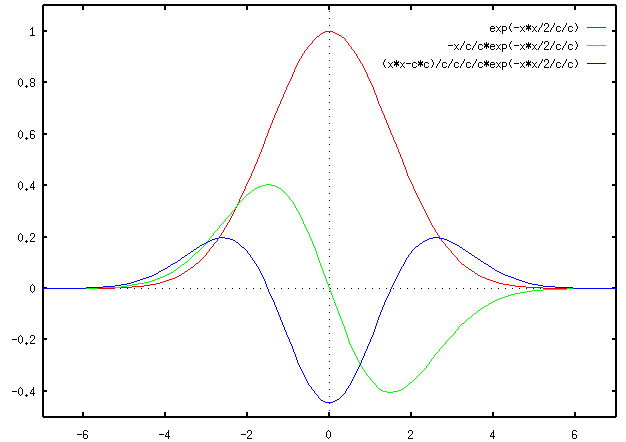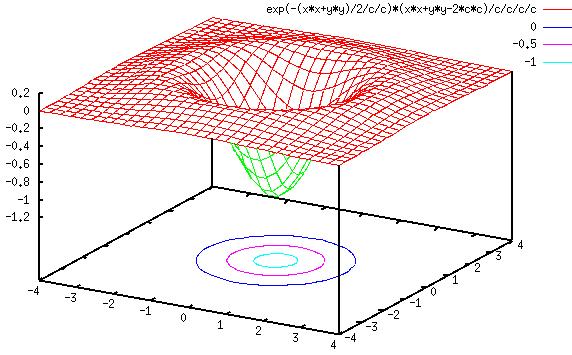二维

5

×

5

5 \times 5

LoG

\text{LoG}

算子：

[

0

0

1

0

0

0

1

2

1

0

1

2

−

16

2

1

0

1

2

1

0

0

0

1

0

0

]

\begin{bmatrix} 0 & 0 & 1 & 0 & 0 \\ 0 & 1 & 2 & 1 & 0 \\ 1 & 2 & -16 & 2 & 1 \\ 0 & 1 & 2 & 1 & 0 \\ 0 & 0 & 1 & 0 & 0 \\ \end{bmatrix}

核矩阵各元素之和必须为零（make sure that the sum (or average) of all elements of the kernel has to be zero）。
边缘检测步骤：
LoG滤波（applying LoG to the image）  过零检测（detection of zero-crossings in the image）  门限判决（threshold the zero-crossings to keep only those strong ones (large difference between the positive maximum and the negative minimum)） 
展开全文• 10.1 Python图像处理之边缘算子-Sobel算子、Roberts算子、拉普拉斯算子、Canny算子、Prewitt算子、高斯拉普拉斯算子 文章目录10.1 Python图像处理之边缘算子-Sobel算子、Roberts算子、拉普拉斯算子、Canny算子、...
10.1 Python图像处理之边缘算子-Sobel算子、Roberts算子、拉普拉斯算子、Canny算子、Prewitt算子、高斯拉普拉斯算子

文章目录
10.1 Python图像处理之边缘算子-Sobel算子、Roberts算子、拉普拉斯算子、Canny算子、Prewitt算子、高斯拉普拉斯算子1 算法原理1.1 Sobel 算子1.2 Roberts 算子1.3 拉普拉斯(Laplacian) 算子1.4 Canny 算法1.5 Prewitt 算子1.6 高斯拉普拉斯算子（LOG 算子）
2 代码3 效果

1 算法原理
图像边缘是图像最基本的特征，所谓边缘(Edge) 是指图像局部特性的不连续性。灰度或结构等信息的突变处称之为边缘。例如，灰度级的突变、颜色的突变,、纹理结构的突变等。边缘是一个区域的结束，也是另一个区域的开始，利用该特征可以分割图像。图像的边缘有方向和幅度两种属性。边缘通常可以通过一阶导数或二阶导数检测得到。一阶导数是以最大值作为对应的边缘的位置，而二阶导数则以过零点作为对应边缘的位置。
边缘检测算子分类：
一阶导数的边缘算子
通过模板作为核与图像的每个像素点做卷积和运算，然后选取合适的阈值来提取图像的边缘。常见的有 Roberts 算子、Sobel 算子和 Prewitt 算子。
二阶导数的边缘算子依据于二阶导数过零点，常见的有 Laplacian 算子，此类算子对噪声敏感。
其他边缘算子
前面两类均是通过微分算子来检测图像边缘，还有一种就是 Canny 算子，其是在满足一定约束条件下推导出来的边缘检测最优化算子。
1.1 Sobel 算子
Sobel 算子是一种用于边缘检测的离散微分算子，它结合了高斯平滑和微分求导。该算子用于计算图像明暗程度近似值，根据图像边缘旁边明暗程度把该区域内超过某个数的特定点记为边缘。Sobel 算子在 Prewitt 算子的基础上增加了权重的概念，认为相邻点的距离远近对当前像素点的影响是不同的，距离越近的像素点对应当前像素的影响越大，从而实现图像锐化并突出边缘轮廓。
Sobel 算子根据像素点上下、左右邻点灰度加权差，在边缘处达到极值这一现象检测边缘。对噪声具有平滑作用，提供较为精确的边缘方向信息。因为 Sobel 算子结合了高斯平滑和微分求导（分化），因此结果会具有更多的抗噪性，当对精度要求不是很高时，Sobel 算子是一种较为常用的边缘检测方法。
Sobel 算子的边缘定位更准确，常用于噪声较多、灰度渐变的图像。其算法模板如下面的公式所示，其中 dx 表示水平方向，dy 表示垂直方向。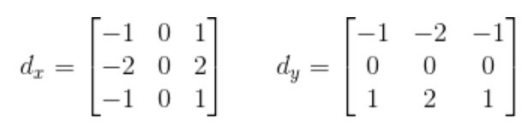1.2 Roberts 算子
Roberts 算子又称为交叉微分算法，它是基于交叉差分的梯度算法，通过局部差分计算检测边缘线条。常用来处理具有陡峭的低噪声图像，当图像边缘接近于正 45 度或负 45 度时，该算法处理效果更理想。其缺点是对边缘的定位不太准确，提取的边缘线条较粗。Roberts 算子的模板分为水平方向和垂直方向，如下式所示，从其模板可以看出，Roberts 算子能较好的增强正负 45 度的图像边缘。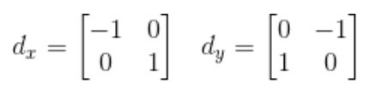在 Python 中，Roberts 算子主要通过 numpy 定义模板，再调用 OpenCV 的 filter2D() 函数实现边缘提取。该函数主要是利用内核实现对图像的卷积运算。
1.3 拉普拉斯(Laplacian) 算子
拉普拉斯(Laplacian) 算子是 n 维欧几里德空间中的一个二阶微分算子，常用于图像增强领域和边缘提取。它通过灰度差分计算邻域内的像素。算法基本流程为：
判断图像中心像素灰度值与它周围其他像素的灰度值，如果中心像素的灰度更高，则提升中心像素的灰度；反之降低中心像素的灰度，从而实现图像锐化操作；  在算法实现过程中，Laplacian 算子通过对邻域中心像素的四方向或八方向求梯度，再将梯度相加起来判断中心像素灰度与邻域内其他像素灰度的关系；  最后通过梯度运算的结果对像素灰度进行调整。
1.4 Canny 算法
Canny 算法是一种被广泛应用于边缘检测的标准算法，其目标是找到一个最优的边缘检测解或找寻一幅图像中灰度强度变化最强的位置。最优边缘检测主要通过低错误率、高定位性和最小响应三个标准进行评价。Canny 算子的简要步骤如下：
去噪声：应用高斯滤波来平滑图像，目的是去除噪声；  梯度：找寻图像的梯度；  非极大值抑制：应用非最大抑制技术来过滤掉非边缘像素，将模糊的边界变得清晰。该过程保留了每个像素点上梯度强度的极大值，过滤掉其他的值；  应用双阈值的方法来决定可能的（潜在的）边界；  利用滞后技术来跟踪边界。若某一像素位置和强边界相连的弱边界认为是边界，其他的弱边界则被删除。
1.5 Prewitt 算子
Prewitt 算子是一种图像边缘检测的微分算子，其原理是利用特定区域内像素灰度值产生的差分实现边缘检测。由于 Prewitt 算子采用 33 模板对区域内的像素值进行计算，而 Robert 算子的模板为 22，故 Prewitt 算子的边缘检测结果在水平方向和垂直方向均比 Robert 算子更加明显。Prewitt 算子适合用来识别噪声较多、灰度渐变的图像，其计算公式如下所示：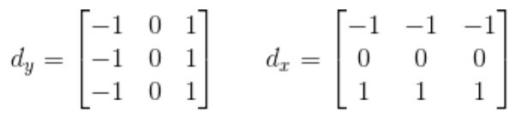在 Python 中，Prewitt 算子的实现过程与 Roberts 算子比较相似。通过 Numpy定义模板，再调用 OpenCV 的 filter2D() 函数实现对图像的卷积运算，最终通过 convertScaleAbs() 和 addWeighted() 函数实现边缘提取。
1.6 高斯拉普拉斯算子（LOG 算子）
高斯拉普拉斯算子（LOG 算子）：是高斯和拉普拉斯的双结合，即集平滑和边沿于一身的算子模型。LoG 算子是由拉普拉斯算子改进而来。拉普拉斯算子是一个单纯的二阶导数算子，是一个标量，具有线性、位移不变性，其传函在频域空间的原点为 0。所有经过拉普拉斯算子滤波的图像具有零平均灰度。但是该算子的缺点是 对噪声具有无法接受的敏感性，因此在实际应用中，一般先要对图像进行平滑滤波，再用拉氏算子进行图像的边缘检测。这就是 LOG 算子的产生的背景（最后的梯度表达式为 高斯函数和原图像卷积，再去二阶微分算子）。以 0 为中心，高斯标准差为 σ 的二维的 LOG 函数的表达式如下所示：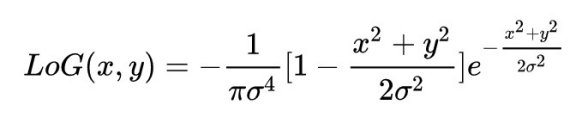2 代码

运行代码说明
1.要改变代码中的图片地址（地址不能有中文）
更改put(path)函数中的路径put(r'../image/image1.jpg')
2.注意最后的plt.savefig('1.new.jpg')是保存plt图像，如果不使用可以注释掉
代码依赖包：
matplotlib  3.4.2
numpy  1.20.3
opencv-python  4.1.2.30

# pip安装
pip install matplotlib numpy opencv-python


import cv2
import numpy as np
import matplotlib.pyplot as plt

def put(path):
# 读取图像
b, g, r = cv2.split(img)
img2 = cv2.merge([r, g, b])

# 灰度化处理图像
grayImage = cv2.cvtColor(img, cv2.COLOR_BGR2GRAY)

# 高斯滤波
gaussianBlur = cv2.GaussianBlur(grayImage, (3, 3), 0)

# 二值化
ret, binary = cv2.threshold(grayImage, 127, 255, cv2.THRESH_BINARY)

# Sobel算子
x = cv2.Sobel(grayImage, cv2.CV_16S, 1, 0)  # 对x求一阶导
y = cv2.Sobel(grayImage, cv2.CV_16S, 0, 1)  # 对y求一阶导
absX = cv2.convertScaleAbs(x)
absY = cv2.convertScaleAbs(y)
Sobel = cv2.addWeighted(absX, 0.5, absY, 0.5, 0)

# Roberts算子
kernelx = np.array([[-1, 0], [0, 1]], dtype=int)
kernely = np.array([[0, -1], [1, 0]], dtype=int)
x = cv2.filter2D(grayImage, cv2.CV_16S, kernelx)
y = cv2.filter2D(grayImage, cv2.CV_16S, kernely)
# 转uint8
absX = cv2.convertScaleAbs(x)
absY = cv2.convertScaleAbs(y)
Roberts = cv2.addWeighted(absX, 0.5, absY, 0.5, 0)

# 拉普拉斯算子
dst = cv2.Laplacian(grayImage, cv2.CV_16S, ksize=3)
Laplacian = cv2.convertScaleAbs(dst)

# 高斯滤波降噪
gaussian = cv2.GaussianBlur(grayImage, (5, 5), 0)

# Canny算子
Canny = cv2.Canny(gaussian, 50, 150)

# Prewitt算子
kernelx = np.array([[1, 1, 1], [0, 0, 0], [-1, -1, -1]], dtype=int)
kernely = np.array([[-1, 0, 1], [-1, 0, 1], [-1, 0, 1]], dtype=int)
x = cv2.filter2D(grayImage, cv2.CV_16S, kernelx)
y = cv2.filter2D(grayImage, cv2.CV_16S, kernely)
# 转uint8
absX = cv2.convertScaleAbs(x)
absY = cv2.convertScaleAbs(y)
Prewitt = cv2.addWeighted(absX, 0.5, absY, 0.5, 0)

# 高斯拉普拉斯算子
gaussian = cv2.GaussianBlur(grayImage, (3, 3), 0)  # 先通过高斯滤波降噪
dst = cv2.Laplacian(gaussian, cv2.CV_16S, ksize=3)  # 再通过拉普拉斯算子做边缘检测
LOG = cv2.convertScaleAbs(dst)

# 用来正常显示中文标签
plt.rcParams['font.sans-serif'] = ['SimHei']

# 显示图形
plt.subplot(241), plt.imshow(img2), plt.title('原始图像'), plt.axis('off')
plt.subplot(242), plt.imshow(binary, plt.cm.gray), plt.title('二值图'), plt.axis('off')
plt.subplot(243), plt.imshow(Sobel, plt.cm.gray), plt.title('Sobel算子'), plt.axis('off')
plt.subplot(244), plt.imshow(Roberts, plt.cm.gray), plt.title('Roberts算子'), plt.axis('off')
plt.subplot(245), plt.imshow(Laplacian, plt.cm.gray), plt.title('拉普拉斯算子'), plt.axis('off')
plt.subplot(246), plt.imshow(Canny, plt.cm.gray), plt.title('Canny算子'), plt.axis('off')
plt.subplot(247), plt.imshow(Prewitt, plt.cm.gray), plt.title('Prewitt算子'), plt.axis('off')
plt.subplot(248), plt.imshow(LOG, plt.cm.gray), plt.title('高斯拉普拉斯算子'), plt.axis('off')

# plt.savefig('1.new-2.jpg')
plt.show()

# 图像处理函数，要传入路径
put(r'../image/image3.jpg')

3 效果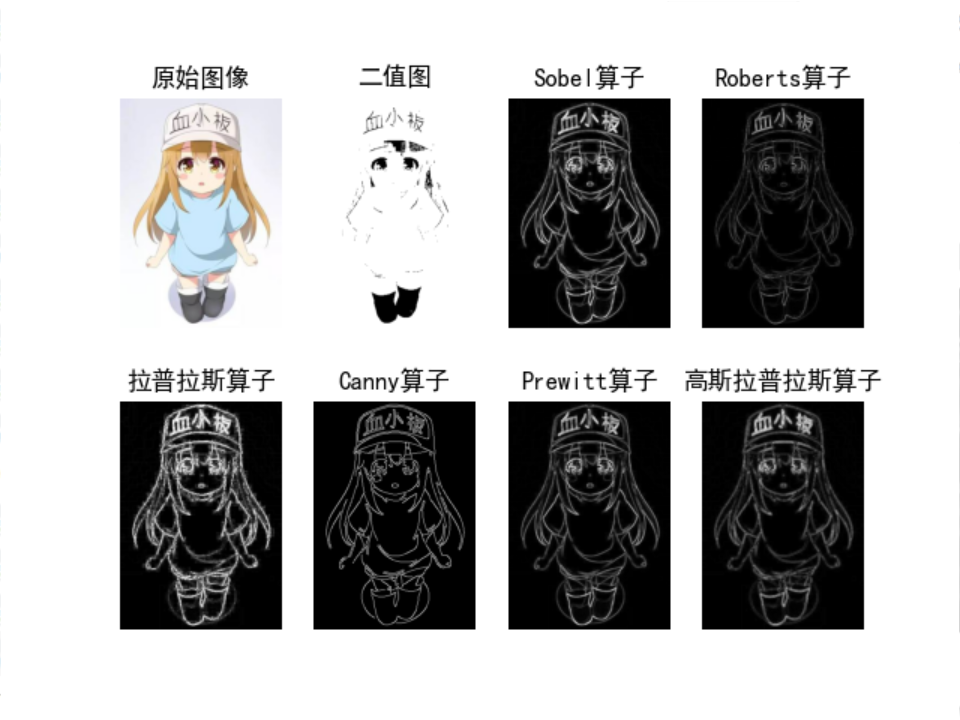展开全文python 算法 图像处理
• Laplacian（拉普拉斯）是对于一张图像的二阶空间导数上各向同性的测量。一张图像的Laplacian会显示出intensity（亮度） 剧烈变化的区域，所以经常用作边缘检测。 一幅图像的拉普拉斯变换可以用下面的式子表示: I(x,...


Laplacian（拉普拉斯）是对于一张图像的二阶空间导数上各向同性的测量。一张图像的Laplacian会显示出intensity（亮度） 剧烈变化的区域，所以经常用作边缘检测。
一幅图像的拉普拉斯变换可以用下面的式子表示: I(x,y)代表带有亮度信息的图像。

因为输入的图像都是用离散的像素表示的，例如256*256，所以我们需要找到离散的卷积核来近似laplacian变换。两个最常用的小卷核是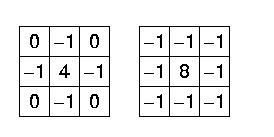因为卷积核是对图像二阶求导的一种近似，所以它对于噪声来说更加敏感。为了克服这一点，通常在进行laplacian之前，先进行Gaussian Smooth(高斯平滑)。所以L*(G*I)， 这里I 表示的是图像，G表示的是Gaussian Smoothing Filter
2D图像中各向同性的高斯形式：

因为卷积运算满足结合律，所以可以先对Gaussian Smoothing Filter 和 Laplacian Filter进行卷积，之后在将卷积结果和图像I 进行卷积。这样一来有两个好处：
（1）两个Filter都是比较小，所以运算少
（2）在对图像进行实时处理时，只有一个卷积运算
到现在为止就变成了使用Laplacian of Gaussian 对图像I 进行卷积， 2-D的LOG（中心在零点，标准差为sigma）如图所示：
2D LOG公式：
这个公式是直接对上文中提到的2D高斯函数进行Laplacian得到的。
和   ，这里推导一下。纯手动敲打，有错的话留言提醒一下。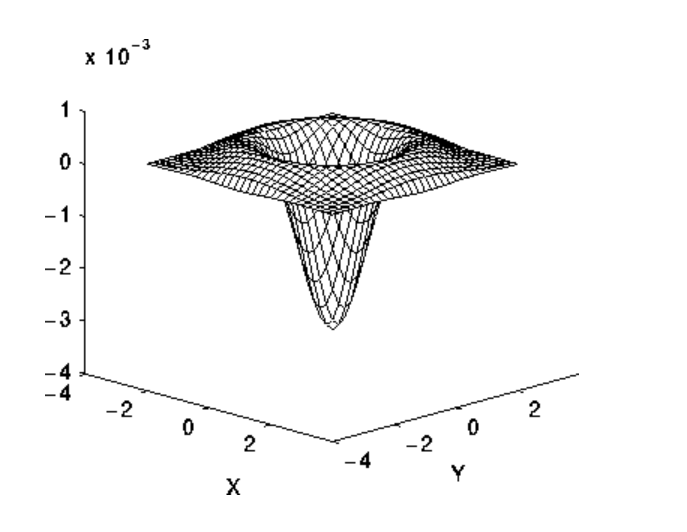根据上面的叙述，对于一个intensity变化剧烈的边界（如下图左，在x左侧比较暗，在x的右侧比较亮）对其进行二阶求导之后，可以发现，x的左侧LOG结果为正，在右侧为负，所以在亮的地方LOG响应为负，在暗的地方响应为正。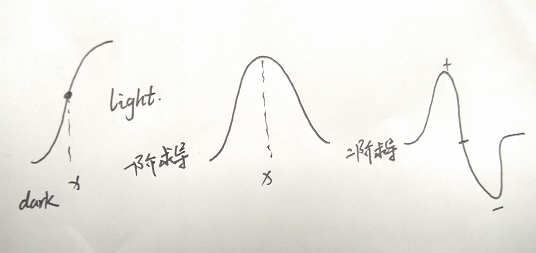所以在边缘处的LOG响应具有如下的特点：
zero at a long distance from the edge(我画的可能不太明显)；positve just to one side of the edge；negative just to the other side of the edge；zero at some point in between, on the edge itself。
参考链接：
https://homepages.inf.ed.ac.uk/rbf/HIPR2/log.htm
https://homepages.inf.ed.ac.uk/rbf/HIPR2/gsmooth.htm


展开全文• 高斯拉普拉斯算子(LoG)算子边缘检测 高斯拉普拉斯算子(LoG)算子边缘检测 1. 前言 2. 高斯拉普拉斯算子(LoG)算子描述 3. 代码实现 1. 前言 在图像中，灰度或结构等信息的突变处称为边缘。边缘可以看作一个区域...
高斯拉普拉斯算子(LoG)算子边缘检测

高斯拉普拉斯算子(LoG)算子边缘检测

1. 前言
2. 高斯拉普拉斯算子(LoG)算子描述
3. 代码实现

1. 前言
在图像中，灰度或结构等信息的突变处称为边缘。边缘可以看作一个区域的结束，另一个区域的开始。利用边缘的特征，可以对图像进行分割。根据定义可以知道，利用各种算法检测到的边缘，并不代表目标的实际边缘。由于图像是二维的，而目标实物是三维的，从三维到二维的投影，已经造成了信息的丢失，再加上成像过程受光照、噪声的影响，使得有边缘的地方不一定被检测出来，而检测出来的边缘也不一定代表实际边缘。 图像的边缘有方向和幅度两个属性，沿边缘方向像素变化平缓，垂直于边缘方向像素变化剧烈。因此，利用图像边缘的变化特性，通过微分算子可以将边缘检查出来，通常用一阶或二阶导数来检测边缘。一阶导数以最大值对应边缘位置，二阶导数以过零点为边缘位置。
展开全文LoG边缘算子 边缘检测 计算机视觉 人工智能
• 说明及程序： 1) 检测特征：使用高斯拉普拉斯算子 (LOG) 公式的尺度空间最大化估计的最稳定的哈里斯点。 使用函数“Harris_Laplace_fn.m”进行估算2) 使用 VLFEAT 将 SIFT 描述符与识别的角点相关联。 3）使用SIFT...matlab
• 边缘检测VS2017编译通过，可直接运行 包括 Roberts边缘算子 Sobel边缘检测算子 Prewitt边缘检测算子 Kirsch边缘检测算子 拉普拉斯算子 高斯拉普拉斯算子
• Dog(Difference of Gaussian)高斯差分 常用于边缘检测、特征检测，这里的Gaussian和高斯低通滤波器的高斯一样，是一个函数，即为正态分布函数，在某一尺度上的特征检测可以通过对两个相邻高斯尺度空间的图像相减，...
• 提出了一种基于高斯拉普拉斯（LoG）算子的加权引导图滤波立体匹配算法。采用加权绝对差与梯度相融合的方式计算匹配代价。利用基于LoG算子的引导图滤波进行代价聚合，使惩戒参数能够随图像中的不同纹理区域进行自适应...
• 该包通过对由高斯滤波器（即 LoG）平滑的图像使用二阶导数来检测边缘。matlab
• 1、拉普拉斯算子的出发点 在图像中，边缘可以看做是位于一阶导数较大的像素处，因此，我们可以求图像的一阶导数来确定图像的边缘，像sobel算子等一系列算子都是基于这个思想的。如下图a表示函数在边沿的时候关系，...
• 基于高斯拉普拉斯算子与自适应优化伽柏滤波的虹膜识别研究论文
• 针对现有梯度算子在图像边缘检测中存在的对噪声比较敏感的问题，提出了一种改进的高斯拉普拉斯算子的边缘检测方法。噪声图像中的边缘检测是一项关键任务，然而目前常用的几种梯度算子，包括已经提出的高斯—...
• Laplacian of Gaussian Operator是用于边缘检测的算子, 其形状如一个墨西哥草帽。 相关的资料很多，本文不赘述，这里谈谈它的卷积模板，常用的卷积模板有 这两个模板是怎么得出的呢？ 经过一番调查，得出一些结论...
• 一、概述 如果图像灰度变化剧烈，进行一阶微分则会形成一个局部的极值，由数学上的知识，对图像进行二阶微分则会形成一个过零点，并且在零点两边...2. Laplace算子是各项同性的，即具有旋转不变性（后面会证明），...
• 高斯拉普拉斯（Laplace of Gaussian） kezunhai@gmail.com http://blog.csdn.net/kezunhai  Laplace算子作为一种优秀的边缘检测算子，在边缘检测中得到了广泛的应用。该方法通过对图像求图像的二阶...
• 拉普拉斯算子是图像二阶空间导数的二维各向同性测度。拉普拉斯算子可以突出图像中强度发生快速变化的区域，因此常用在边缘检测任务当中。在进行Laplacian操作之前通常需要先用高斯平滑滤波器对图像进行平滑处理，以...
• 物体的边缘是图像局部变化的重要特征,以不连 续性的形式出现,通常用方向和幅度描述图像的边缘 特性。一般来讲,沿边缘走向的像素变换平缓,而垂 直于边缘走向的像素变化剧烈。基于边缘检测的基 本思想是先检测图像中的...
• 来自为知笔记(Wiz) 转载于:https://www.cnblogs.com/fireae/p/3699037.html
• 一、简要描述：拉普拉斯算子是图像二阶空间导数的二维各向同性测度。拉普拉斯算子可以突出图像中强度发生快速变化的区域，因此常用在边缘检测任务当中。在进行Laplacian操作之前通常需要先用高斯平滑滤波器对图像...
• 1、拉普拉斯算子的出发点 在图像中，边缘可以看做是位于一阶导数较大的像素处，因此，我们可以求图像的一阶导数来确定图像的边缘，像sobel算子等一系列算子都是基于这个思想的。如下图a表示函数在边沿的时候关系，...LOG
• 这是对图像的基础处理，其中包含了对图像进行FFT，频谱中心化，分别用高斯低通，高斯高通在频域处理图像以及在频域用拉普拉斯算子对图像进行锐化matlab
• 拉普拉斯算子 # 图像增强算法 # 拉普拉斯算子，自编写实现，利用空域卷积运算实现滤波 # 具有图像增强效果，同时亦可用于边缘检测、角点检测 import cv2 import numpy as np import matplotlib.pyplot as plt if ...python 边缘检测
• 请用MATLAB或其它语言编写相关程序，输入一幅灰度图像，分别采用梯度算子、拉普拉斯算子、Sobel算子、Prewitt算子对图像进行锐化，在同一个窗口输出显示原始图像和４种结果图像。...